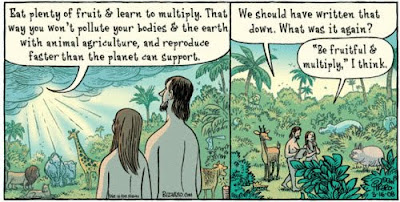## Tuesday, March 8, 2011

### Eat fruit and understand exponential growth!

You still believe Malthus was wrong? Study in a social "sciences" department, did you?Exponential growth is counter-intuitive, but only very simple math is required. The "rule of 69" tells us that with a constant rate of growth, the doubling time (T2) is 69 years divided by the annual growth rate (in percent): ln2/k ≈ 0.69/k. With k the annual rate of growth, in percent, the doubling time, in years, is approximately 69/k. (With k=0.02 or 2% (2/100), the doubling time is ln2/k≈100*0.69/2≈35 years; If something grows steadily at 7% per year (k=0.07), the doubling time is 69/7≈10 years). This is all easily derived from the exponential growth function r(t)=r(0)exp(kt), where r(t) is the quantity r at time t, r(0) is the quantity at the start (t=0), and k is the growth rate. The doubling time is the time, T2, at which r(T2)=2r(0), that is, 2r(0)=r(0)exp(k(T2)), which implies that T2= ln2/k ≈0.69/k.

Furthermore, with each successive doubling, the resulting quantity is greater than the cumulative total of all quantities before that since the process began.

The quantity in question can be money, a particular resource, or an animal population, such as the human population size—anything that is capable of growing (for a while) at a constant rate of growth.

If r is a resource, such as global oil reserves, suppose we have R tons of oil left, and we are increasing the rate of oil use by 100k% per year. The expiration time, Te, the time it takes to use up all that oil, is the amount of time till the total used is equal to R. The total resource used by time T, is the integral from t=0 to T of r(t) with respect to t, which is ∫r(t)dt=∫r(0)exp(kt)dt=r(0)(exp(kT)-1)/k. Hence the expiration time, Te, is where R = r(0)(exp(kTe)-1)/k, so Te= ln((kR/r(0))+1)/k.

An excellent introduction to exponential growth can be found here:

You can watch Dr. Bartlett's entire lecture, piecemeal, here, or here.

Do it! It is every voting citizen's duty to acquire the understanding and the prerequisites needed to comprehend the important issues of our time.

If you are only going to watch one 9 minute segment of the lecture, part 3 gives a particularly vivid example:

It doesn't matter whether the growth is in population or in consumption.

Money can grow indefinitely, because the money we use today is simply debt. There is no absolute limit to the total amount of debt humanity can have. But there is a limit to the stuff we can buy with that money (debt)... Thor believes you can figure out the consequences of that for yourself...

"Woe unto them that join house to house, that lay field to field, till there be no place…"
—Isaiah 23, 005:008